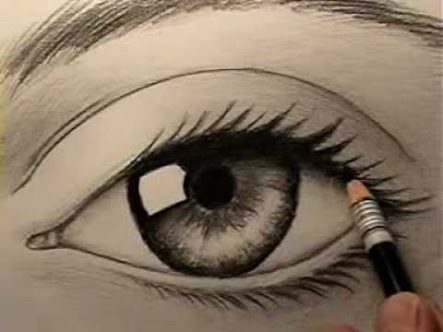# Problems With Everyday Objects: The Human Eye

Geometry Level 5Above (constricted pupil)

Below (dilated pupil)Below (Geometric Representation$~~~$ The human eye is a very beautiful and delicate part of our body.

$~~~$ It can show joy, sadness, boredom, and confidence with out making a sound.

$~~~$ When looking upon a loved one, the pupil (the black small circle in your eye) tends to dilate (increase in size). However, when we look at something that we feel indifferent to or bored of, such as the white board in history class, our pupils tend to constrict (decrease in size). Fun fact: the ratio of the width of the human head to the distance between your eyes tends to be around 1.618, or the golden ratio.

$~~~$ When fully dilated, the radius of the eye can be up to 4 as large as when fully constricted.

$~~~$ Depicted above are pictures of a constricted pupil, dilated pupil, and a geometric representation of two eyes, one constricted and one dilated.

$~~~$ The red and green lines are tangent to the black circles (pupils) and blue circles (irises) respectively. The left pupil is fully constricted and the right pupil is fully dilated (meaning it's radius is 4 times as large as the left one). The ratio of the radius of the constricted pupil to the radius of the entire left circle is 1:5. Finally, if the length of the red segment is $7\sqrt{59}$ and the length of green segment is $16\sqrt{11}$, find the radius of the large black circle (dilated pupil).

DO NOT TRY TO WORK WITH THE TWO NUMBERS THAT HAVE RADICALS.

Instead, begin to work with the given ratios and generalize two formulas to create a system of two equations

This problem is actually rather elegant, thus use of a computer program is not allowed, although you may use a calculator for the operations PEMDAS. Hint: the distance between the circles is integral.

All the numbers used in this problem are in millimeters. They are almost at a 1:1 scale with an actual human face.

×